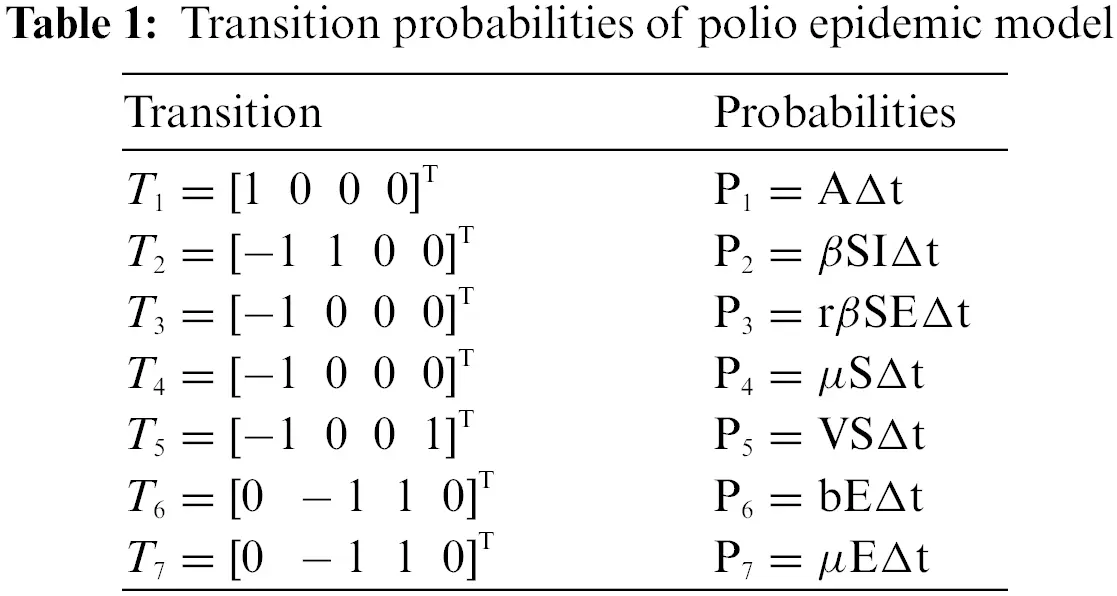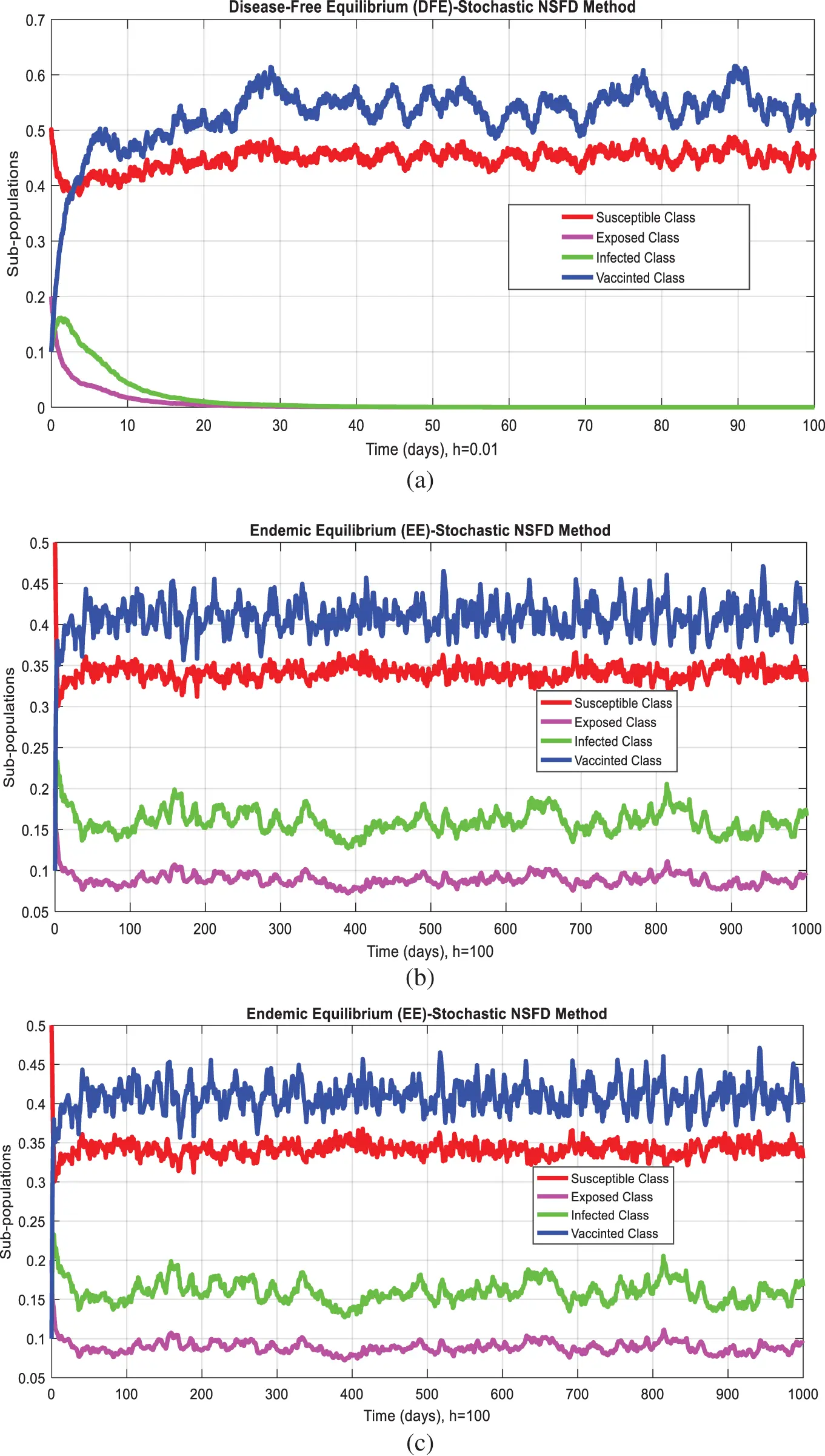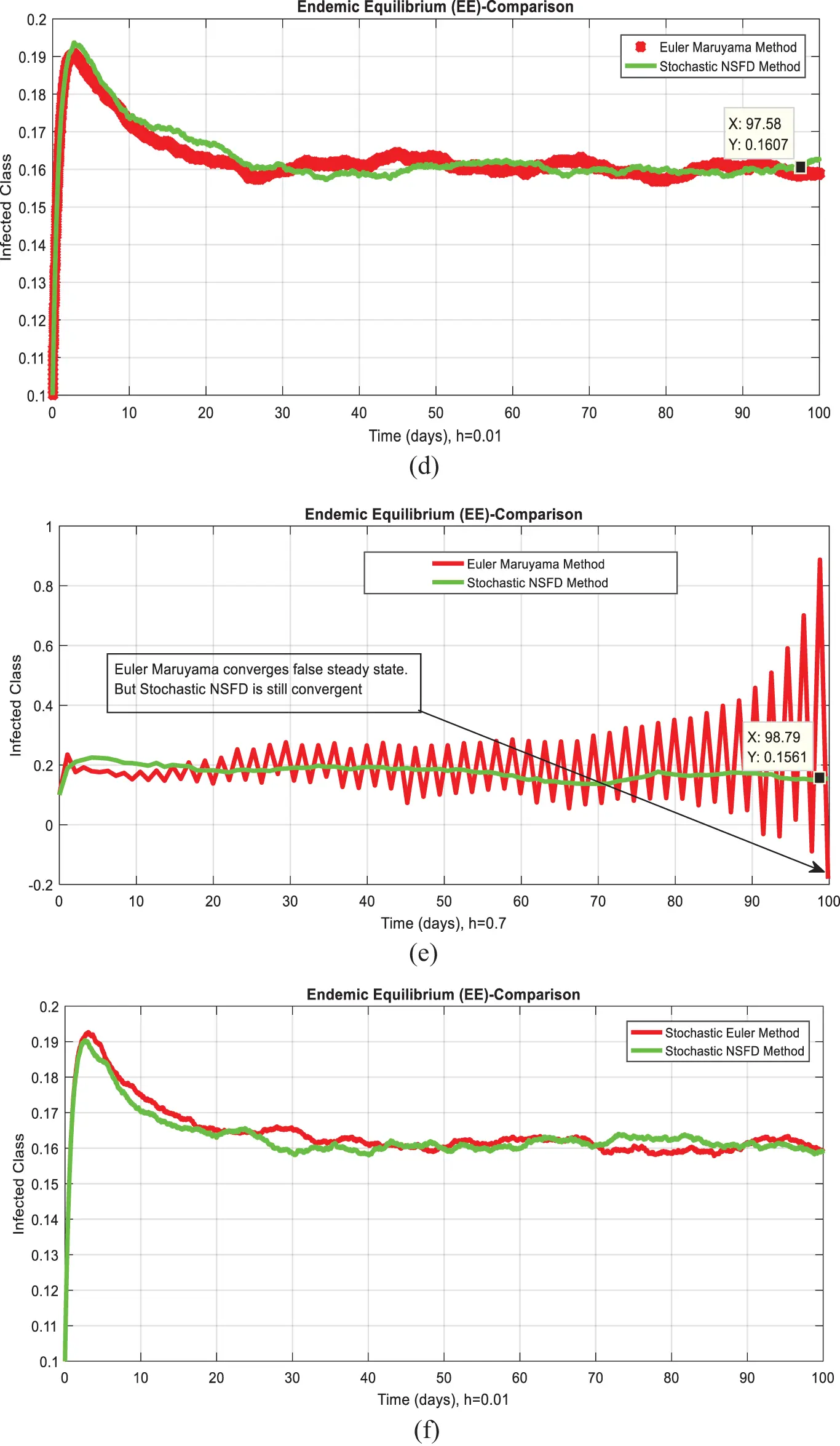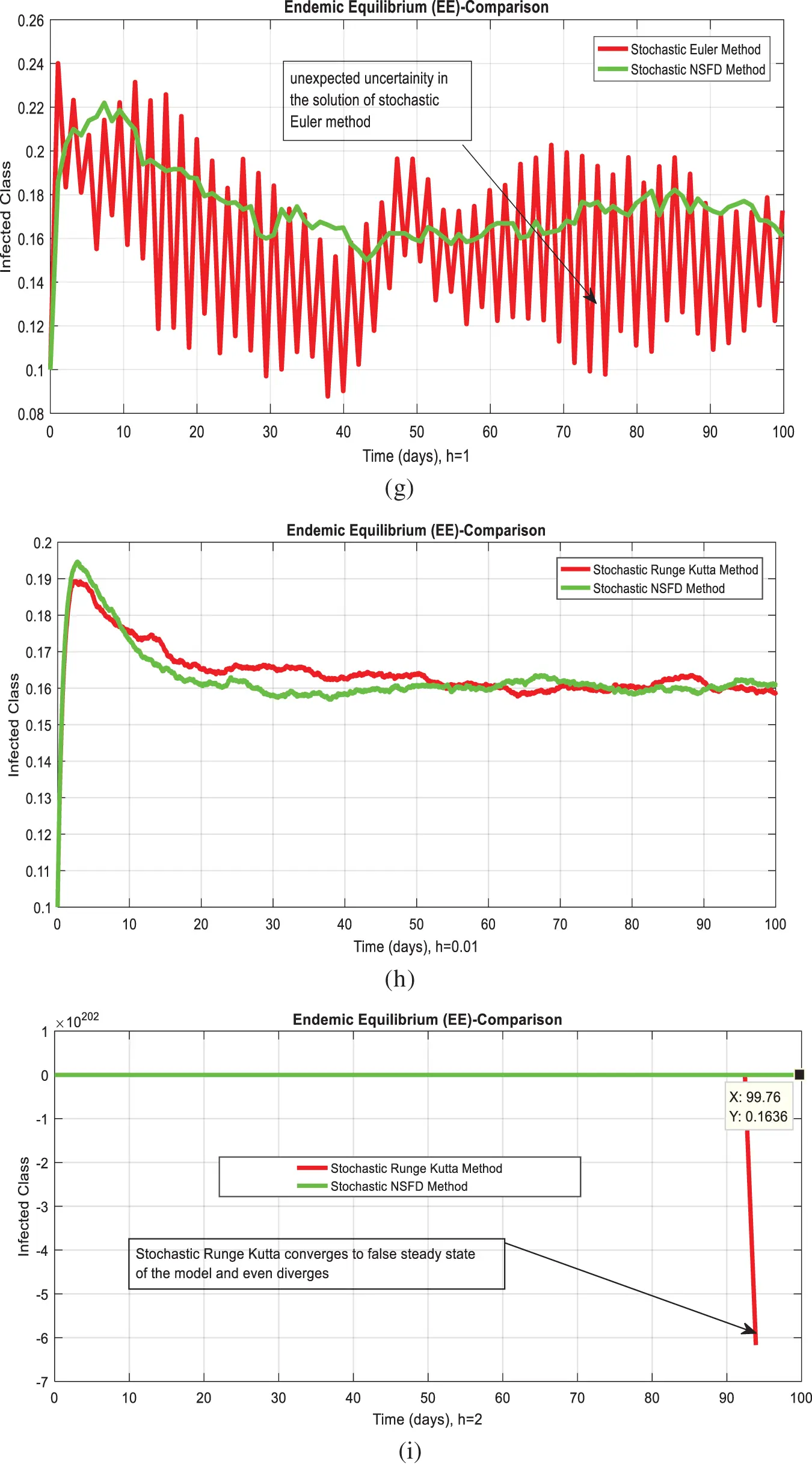## Table of ContentOpen Access

ARTICLE

# Stochastic Analysis for the Dynamics of a Poliovirus Epidemic Model

Ali Raza1, Dumitru Baleanu2,3,4, Zafar Ullah Khan5, Muhammad Mohsin6,*, Nauman Ahmed7, Muhammad Rafiq8, Pervez Anwar9

1 Department of Mathematics, Government Maulana Zafar Ali Khan Graduate College Wazirabad, Punjab Higher Education Department (PHED), Lahore, 54000, Pakistan
2 Department of Mathematics, Cankaya University, Balgat, Ankara, 06530, Turkey
3 Department of Medical Research, China Medical University, Taichung, 40402, Taiwan
4 Institute of Space Sciences, Magurele-Bucharest, 077125, Romania
5 Department of Dermatology, Rashid Latif Medical College Lahore, Lahore, 54000, Pakistan
6 Department of Mathematics, Technische Universitat Chemnitz, Chemnitz, 6209111, Germany
7 Department of Mathematics and Statistics, The University of Lahore, Lahore, 54590, Pakistan
8 Department of Mathematics, Faculty of Science and Technology, University of Central Punjab, Lahore, 54000, Pakistan
9 Department of Biochemistry, University of Sialkot, Sialkot, 51311, Pakistan

* Corresponding Author: Muhammad Mohsin. Email:(This article belongs to this Special Issue: Bio-inspired Computer Modelling: Theories and Applications in Engineering and Sciences)

Computer Modeling in Engineering & Sciences 2023, 136(1), 257-275. https://doi.org/10.32604/cmes.2023.023231

## Abstract

Most developing countries such as Afghanistan, Pakistan, India, Bangladesh, and many more are still fighting against poliovirus. According to the World Health Organization, approximately eighteen million people have been infected with poliovirus in the last two decades. In Asia, still, some countries are suffering from the virus. The stochastic behavior of the poliovirus through the transition probabilities and non-parametric perturbation with fundamental properties are studied. Some basic properties of the deterministic model are studied, equilibria, local stability around the stead states, and reproduction number. Euler Maruyama, stochastic Euler, and stochastic Runge-Kutta study the behavior of complex stochastic differential equations. The main target of this study is to develop a nonstandard computational method that restores dynamical features like positivity, boundedness, and dynamical consistency. Unfortunately, the existing methods failed to fix the actual behavior of the disease. The comparison of the proposed approach with existing methods is investigated.

## Keywords

1  Introduction

Jenkins et al. in 2006 formulated a model in which he concluded that the use of monovalent is better than other vaccines. It provides outstanding outbreak control . Haldar et al.  introduced the poliovirus vaccine in India. Kalkowska et al. in 2020 represent a differential equation-based stochastic model for poliovirus transmission. The model shows the poliovirus transmission for 2019 to 2023 with a strategic eradication plan . Minor studied the types of polioviruses, vaccination, and eradication of the virus worldwide . Thompson  investigated the transmission dynamics of the poliovirus in Nigeria. Duque-Marin et al.  studied two types of vaccines in the mathematical model. Denes et al.  presented a model which describes polio transmission in tropical regions. Cheng et al.  discussed a polio vaccination model in two different age classes. Alba et al.  addressed the correlation between climate and vaccination through a mathematical model. Shaghaghi et al. in 2018, studied that the OPV and IVPPvs vaccine was helpful for the eradication of the virus last few years . Shimizu in 2014  explained IPV is very effective against the poliovirus, and the author reviewed the introduction, development, and characterization of the OPV vaccine. In addition, his place in the world was told. Rafique et al. in 2020 presented a mathematical model in which they discovered the dynamics of poliovirus transmission using standard methods with vaccination . Nidia et al.  in 2007, examined the effects of the poliovirus on human life and the steps taken to eradicate the virus and discussed what steps we could take in the future to get rid of it. Thompson et al.  presented polio outbreaks in the USA. Kalkowska et al. introduced a model to identify poliovirus and opportunities to increase population immunity . Kim et al.  presented a model to examine the transmission of virulent circulating vaccine-derived polioviruses. Hillis  formulated a model in different regions before using artificial poliovirus vaccination. Mendrazitsky et al.  explained a disease of epidemic development model. The model analyzed other properties of polio and its non-equilibrium outbreak dynamics. Debanne et al.  presented a mathematical model of poliovirus in America. Naik et al. [20,21] studied the fractional modeling of cancer and HIV infection with the well-known results of stabilities.

The strategy of the paper is as follows. The first section goes to literature, and Section 2 goes to stochastic modeling of poliovirus and its fundamental properties. Section 3 goes to the proposed numerical method and its simulation with current approaches in the literature. Section 4 goes to the paper’s conclusion and remarks.

2  Poliovirus Model

For any time t, S: represents the class that is influenced by infection, E: represents the class that is disclosed by infection, I: represents an infective class, V: represents immunization class, A: represents the constant immigration rate of the human population. β: is the per unit time probability of infection transmission by the infective population. r: is the reduction in the exposed class due to transmission of infection. v: represents the proportion of recruits in the susceptible class moving to the vaccinated class, v1: is the number of vaccinated exposed populations, b: number of exposed populations moving to the infection class. µ: natural death of the human population, α: disease death rate. The first order, nonlinear, and coupled ordinary differential equations of the poliovirus epidemic model are assumed as follows:

dS=(AβSIrβSE(μ+v)S)dt+σ1SdB(t).(1)

dE=(βSI+rβSE(b+μ+v1)E)dt+σ2EdB(t).(2)

dI=((b+v1)E(μ+α)I)dt+σ3IdB(t).(3)

dV=(vSμV)dt+σ4VdB(t).(4)

with initial condition S (0) ≥ 0; E (0) ≥ 0; I (0) ≥ 0; V (0) ≥ 0, and (σi:i=1,2,3,4) is the peutrbation term with B(t) is the Brownian motion [22,23].

2.1 Properties 

This section studies the positivity and boundedness of the system ((1)(4)). Let us consider the vector as follows:

U(t)=(S(t),E(t),I(t),V(t)).(5)

And the norm |U(t)|=S2(t)+E2(t)+I2(t)+V2(t).

dU(t)=H(U,t)dt+K(U,t)dW(t).(6)

As, L=t+i=14Hi(U,t)ui+12i,j=14(KT(U,t)K(U,t))i,j×2UiUj, where L is differential operator.

If L acts on a function V1C2,1(R4×(0,);R+) then we denote

LV(U,t)=V1t(U,t)+V1U(U,t)H(U,t)+12Trace(KT(U,t)VUU(U,t)K(U,t)).

where transportation is denoted by T.

Theorem 1: For model ((1)(4)) and any given initial value (S(0),E(0),I(0),V(0))R+4, there is a unique solution (S(t),E(t),I(t),V(t)) on t0 and will remain in R+4 with probability one.

Proof: By Ito’s formula, the model ((1)(4)) admits a positive solution in the unique local on [0,τe], and explosion time is denoted by τe. Because the local Lipschitz condition is satisfied by all the coefficients of the model as mentioned earlier.

Next, let us show that the given model ((1)(4)) admits this solution in the global sense; that is, τe= almost sure.

Let mo=0 be sufficiently large for S(0),E(0),I(0), and V(0) lying with the interval [1mo,mo]. For each integer mmo, define a sequence that is so-called stopping times as

τm=inf{tϵ[0,τe]:S(t)(1m,m)orE(t)(1m,m)orI(t)(1m,m)orV(t)(1m,m)}.(7)

where we set infϕ=(ϕ represents the empty set). Since τm is non-decreasing as m,

τ=limmτm.(8)

Then ττe. To prove, τ=.

In case of violation of statement, then T>0 and εϵ(0,1) such that

P{τT}>ε.(9)

this, there is an integer m1>m0 such that

P{τmT}ε,mm1.(10)

Define a C4 function V:R+4R+ by

V1(S,E,I,V)=(S1lnS)+(E1lnE)+(I1lnI)+(V1lnV).(11)

By using Ito’s formula, we calculate

dV1(S,E,I,V)=(11S)dS+(11E)dE+(11I)dI+(11V)dV+σ22dt.

dV1(S,I,R)=(11S)((AβSIrβSE(μ+v)S)dt+σ1SdB(t))+(11E)×((βSI+rβSE(b+μ+v1)E)dt+σ2EdB(t))+(11I)×(((b+v1)E(μ+α)I)dt+σ3IdB(t))+(11R)[(vSμV)dt+σ4VdB(t)]dt+σ22dt.

dV1(S,E,I,V)[A+μ+v+α+σ22]dt+σ1SdB(t)++σ2EdB(t)+σ3IdB(t)++σ4VdB(t).(12)

Let, N=A+μ+v+α+σ22, Then Eq. (12) could be written as

dV1(S,E,I,V)Ndt+σ1SdB(t)++σ2EdB(t)+σ3IdB(t)++σ4VdB(t).(13)

By integrating from 0 to τmτ, we get

0τmτdV1(S(s),E(s),I(s),V(s))0τmτNds+0τmτ(σ1S+σ2E+σ3I+σ4V)dB(s).(14)

where τmτ=min(τm,T), the taking the expectations to lead to

EV1(S(τmτ),I(τmτ),E(τmτ),V(τmτ))V(S(0),E(0),I(0),V(0))+NT(15)

Set Ωm={τmT} for m>m1, and from (15), we have P(Ωmε). For every vΩm there are some i such that ui(τm,v) equals either m or 1m for i=1,2,3;

Hence, V1(S(τm,v),E(τm,v),I(τm,v),V(τm,v)) is less than min{m1lnm,1m1ln1m}.

Then we obtain

V1(S(0),E(0),I(0),V(0))+NTE(IΩm(v)V1(S(τm),E(τm),I(τm),V(τm))){min(m1lnm,1m1ln1m)}.(16)

IΩm(v) of Ωm represents the indicator function. Letting m leads to the contradiction =V1(S(0),E(0),I(0),V(0))+NT<.

As desired.

2.2 Equilibria of Model

The disease-free equilibrium of the model is 0=(Aμ+v,0,0,vμS).

The endemic equilibrium of the model is denoted by K1 = (S*, E*, I*, V*).

S=(b+μ+v1)(μ+α)β(b+v1)+rβ(μ+α), E=A(μ+v)SrβS+βS(b+v1μ+α), I=b+v1μ+αE, and V=vμS.

2.3 Local Stability of Model

Theorem 2: The disease-free equilibrium 0=(Aμ+v,0,0,vμS) is locally asymptotically stable if R0<1; otherwise, unstable if R0>1.

Proof: Considering the function from the system ((1)(4)) as follows:

F1=AβSIrβSE(μ+v)S.

F2=βSI+rβSE(b+μ+v1)E.

F3=(b+v1)E(μ+α)I.

F4=vSμV.

The elements of the given Jacobean Matrix at KO is as follows:

J(KO)=[(μ+v)rβAμ+VβAμ+V00rβAμ+V(b+μ+v1)βAμ+V00b+v1(μ+α)0V00μ].

J(KOλI)=|(μ+v)λrβAμ+VβAμ+V00rβAμ+V(b+μ+v1)λβAμ+V00b+v1(μ+α)λ0V00μλ|=0.

(μλ)|(μ+v)λrβAμ+VβAμ+V0rβAμ+V(b+μ+v1)λβAμ+V0b+v1(μ+α)λ|=0.

λ1=μ<0,

|(μ+v)λrβAμ+VβAμ+V0rβAμ+V(b+μ+v1)λβAμ+V0b+v1(μ+α)λ|=0.

(μ+v)λ|rβAμ+V(b+μ+v1)λβAμ+Vb+v1(μ+α)λ|=0.

λ2=(μ+v)<0.

λ2+λ[(μ+α)rβAμ+v+λ2]rβAμ+v(μ+α)βAμ+v(b+v1)=0.

λ2+λ[(μ+α)+(b+μ+v1)(1R2)]+(μ+α)(b+μ+v1)(1R0)=0.

We obtain the following results by applying Routh Hurwitz criteria for 2nd order.

A1>0,A2>0, if R0,R2<1, where A1=[(μ+α)+(b+μ+v1)(1R2)], A2=(μ+α)(b+μ+v1)(1R0).

Theorem 3: The endemic equilibrium K1 = (S*, E*, I*, V*) is locally asymptotically stable if Ro>1.

Proof: The Jacobean matrix at K1=(S,E,I,V) is as follows:

J(K1)=[βIrβE(μ+v)rβSβS0βI+rβErβS(b+μ+v1)βS00b+v1(μ+α)0V00μ].

|J(K1λI)|=|βIrβE(μ+v)λrβSβS0βI+rβErβS(b+μ+v1)λβS00b+v1(μ+α)λ0V00μλ|=0.

(μλ)|βIrβE(μ+v)λrβSβSβI+rβErβS(b+μ+v1)λβS0b+v1(μ+α)λ|=0.

λ1=μ<0,

|βIrβE(μ+v)λrβSβSβI+rβErβS(b+μ+v1)λβS0b+v1(μ+α)λ|=0

βIrβE(μ+v)λ|rβS(b+μ+v1)λβSb+v1(μ+α)λ|(βI+rβE)|rβSβSb+v1(μ+α)λ|=0.

βIrβE(μ+v)λ[(rβS(b+μ+v1)λ)((μ+α)λ)(βS)(b+v1)](βI+rβE)[(rβS)((μ+α)λ)(βS)(b+v1)]=0.

βIrβE(μ+v)λ[rβS(μ+α)rβSλ+(b+μ+v1)(μ+α)+(b+μ+v1)λ+(μ+α)λ+λ2(βS)(b+v1)]βIrβE[rβS(μ+α)+rβSλ+βSb+v1]=0.

rβ2SI(μ+α)+rβ2SIλβI(b+μ+v1)(μ+α)βI(b+μ+v1)λβI(μ+α)λβIλ2+β2SI(b+v1)+r2β2ES(μ+α)+r2β2ESλrβE(b+μ+v1)(μ+α)rβE(b+μ+v1)λrβE(μ+α)λrβEλ2+rβES(b+v1)+rβS(μ+α)(μ+v)+rβS(μ+v)λ(b+μ+v1)(μ+α)(μ+v)(μ+v)(b+μ+v1)λλ(μ+α)(μ+v)(μ+v)λ2+(μ+v)βS(b+v1)+rβS(μ+α)λ+rβSλ2(b+μ+v1)(μ+α)λ(b+μ+v1)λ2(μ+α)λ2λ3+(βS)(b+v1)λrβ2SI(μ+α)rβ2SIλβ2SI(b+v1)r2β2ES(μ+α)r2β2ESλrβ2SE(b+v1)=0.

λ3λ2[βI+rβE+(μ+v)rβS+(b+μ+v1)+(μ+α)]λ[rβ2SI+βI(b+μ+v1)+βI(μ+α)+rβE(b+μ+v1)+rβE(μ+α)rβS(μ+v)+(μ+v)(b+μ+v1)+(μ+α)(μ+v)rβS(μ+α)+(b+μ+v1)(μ+α)(βS)(b+v1)+rβ2SI][rβ2SI(μ+α)+βI(b+μ+v1)(μ+α)r2β2ES(μ+α)+rβE(b+μ+v1)(μ+α)rβES(b+v1)rβS(μ+α)(μ+v)+(b+μ+v1)(μ+α)(μ+v)(μ+v)βS(b+v1)+rβ2SI(μ+α)+r2β2ES(μ+α)+rβ2SE(b+v1)]=0.

[λ3+λ2A+λC+D]=0.

λ3+λ2A+λC+D=0.

where A= βI+rβE+(μ+v)rβS+(b+μ+v1)+(μ+α),

B=rβ2SI+βI(b+μ+v1)+βI(μ+α)+rβE(b+μ+v1)+rβE(μ+α)rβS(μ+v)+(μ+v)(b+μ+v1)+(μ+α)(μ+v)rβS(μ+α)+(b+μ+v1)(μ+α)(βS)(b+v1)+rβ2SI,

C=rβ2SI(μ+α)+βI(b+μ+v1)(μ+α)r2β2ES(μ+α)+rβE(b+μ+v1)(μ+α)rβES(b+v1)rβS(μ+α)(μ+v)+(b+μ+v1)(μ+α)(μ+v)(μ+v)βS(b+v1)+rβ2SI(μ+α)+r2β2ES(μ+α)+rβ2SE(b+v1),

D=rβ2SI(μ+α)+βI(b+μ+v1)(μ+α)r2β2ES(μ+α)+rβE(b+μ+v1)(μ+α)rβES(b+v1)rβS(μ+α)(μ+v)+(b+μ+v1)(μ+α)(μ+v)(μ+v)βS(b+v1)+rβ2SI(μ+α)+r2β2ES(μ+α)+rβ2SE(b+v1).

Applying Routh-Hurwitz Criterion for 3rd order, A>0,D>0, and AC>D, if R0>1.

Hence the given system is locally asymptotically stable.

2.4 Reproduction Number

The idea of reproduction number is presented in  by considering Eqs. (3) and (4), we get the following matrices:

[EIV]=[rSββS0000000][EIV][b+μ+v100bv1μ+α000μ][EIV].

Here, F =[rSββS0000000] and G =[b+μ+v100bv1μ+α000μ]

FG1=[rβA(μ+v)(b+μ+v1)+βA(b+v1)(μ+v)(μ+α)(b+μ+v1)βA(μ+v)(μ+α)0000000].

The spectral radius of the FG1 is called the reproduction number is as follows:

R0=R1+R2

R0=βA(b+v1)(μ+v)(μ+α)(b+μ+v1)+rβA(μ+v)(b+μ+v1)

where R1=βA(b+v1)(μ+v)(μ+α)(b+μ+v1) , R2=rβA(μ+v)(b+μ+v1).

3  Stochastic Poliovirus Epidemic Model

Let us consider the vector C=[S,E,I,V]T of stochastic differential equations (SDEs) of the poliovirus epidemic model ((1)(4)). We want to calculate the expectation and variance (see Table 1).Drift=G(C,t)=[AβSIrβSEμSVSβSI+rβSEbEμEv1EbE+v1EμI+αIAμV]Δt.

Diffusion=[A+βSI+rβSE+μS+vSβSIrβSE0vSβSIrβSEβSI+rβSE+bE+μE+v1EbEv1E00bEv1EbE+v1E+μI+αI0vS00vS+μV]

The equation of poliovirus epidemic model ((1)(4)) can be written as

d[SEIV]=[AβSIrβSEμSVSβSI+rβSEbEμEv1EbE+v1EμI+αIAμV]dt+[A+βSI+rβSE+μS+vSβSIrβSE0vSβSIrβSEβSI+rβSE+bE+μE+v1EbEv1E00bEv1EbE+v1E+μI+αI0vS00vS+μV]dB.(17)

The Euler Maruyama approach is cast-off to determine the numerical result of the Eq. (17) by using the values of the parameters given in Table 2 as follows:Cn+1=Cn+f(Cn,t)Δt+L(Cn,t)dB.

[Sn+1En+1In+1Vn+1]=[SnEnInVn]+[[AβSnInrβSnEnμSnvSnβSnIn+rβSnEnbEnμEnv1EnbEn+v1EnμIn+αInAμVn]]Δt+[A+βSnIn+rβSnEn+μSn+vSnβSnInrβSnEn0vSnβSnInrβSnEnβSnIn+rβSnEn+bEn+μEn+v1EnbEnv1En00bEnv1EnbEn+v1En+μIn+αIn0vSn00vSn+μVn]ΔBn(18)

where C(0)=Co=[0.5,0.3,0.2,0.1,]T,0tC and  Brownian motion is denoted as B.

3.1 Stochastic Nonstandard Finite Difference Method

The stochastic NSFD can be developed for the system ((1)(4)) as

dSdt=AβSIrβSE(μ+v1)S.

The breakdown of the proposed method for the above equation.

Sn+1=Sn+h[AβSn+1InrβSn+1En(μ+v1)Sn+1+σ1SnΔB1].

Sn+1=Sn+hA+hσ1SnΔB11+hβIn+hrβEn+h(μ+v1).(19)

Similarly, we break the remaining system into a proposed method like (19), as follows:

En+1=En+hβSnIn+hrβSnEn+hσ2EnΔB21+h(b+μ+v1).(20)

In+1=In+h((b+v1)En+σ3InΔB31+h(μ+α).(21)

Vn+1=Vn+hv1sn+σ4VnΔB41+hμ.(22)

where, n = 0, 1, 2,…, and discretization gap is denoted by “h”.

3.2 Stability Analysis

Theorem 5: The stochastic NSFD method is stable if the eigenvalues of Eqs. (19)(22) lie in the unit circle for any n0.

Proof: Let the functions L1, L2, L3, L4 by assuming ΔBn=0, from the system ((19)(22)) as follows:

L1=S+hA1+hβI+hrβE+h(μ+v1),L2=E+hβSI+hrβSE1+h(b+μ+v1),L3=I+h((b+v1)E1+h(μ+α),L4=V+hv1S1+hμ.

The elements of the Jacobean matrix are given as

J(S,E,I,V)=[L1SL1EL1IL1VL2SL2EL2IL2VL3SL3EL3IL3VL4SL4EL4IL4V].

The given Jacobean matrix at 0=(Aμ+v,0,0,vμS) is as follows:

J(KO)=[11+h(μ+v1)(Aμ+v+hA)rhβ[1+h(μ+v1)]2hβ(Aμ+v+hβ)[1+h(μ+v1)]20011rhβ(Aμ+v)+h(b+μ+v1)hβ(Aμ+v)(1+rhβ(Aμ+v)+h(b+μ+v1))[1rhβ(Aμ+v)+h(b+μ+v1)]200h(b+v1)1+h(μ+α)00hv11+hμ0011+hμ].

|11+h(μ+v1)λ(Aμ+v+hA)rhβ[1+h(μ+v1)]2hβ(Aμ+v+hβ)[1+h(μ+v1)]20011rhβ(Aμ+v)+h(b+μ+v1)λhβ(Aμ+v)(1+rhβ(Aμ+v)+h(b+μ+v1))[1rhβ(Aμ+v)+h(b+μ+v1)]200h(b+v1)1+h(μ+α)λ0hv11+hμ0011+hμλ|=0.

11+hμλ|11+h(μ+v1)λ(A+hA(μ+v))rhβ(μ+v)[1+h(μ+v1)]2hβAhβ(μ+v)(μ+v)[1+h(μ+v1)]20μ+v(μ+v)rhβA+(μ+v)h(b+μ+v1)λhβA((μ+v)+rhβA+(μ+v)h(b+μ+v1))[(μ+v)rhβA+(μ+v)h(b+μ+v1)]20h(b+v1)1+h(μ+α)λ|=0

λ1=11+hμ<1,

|11+h(μ+v1)λ(A+hA(μ+v))rhβ(μ+v)[1+h(μ+v1)]2hβAhβ(μ+v)(μ+v)[1+h(μ+v1)]20μ+v(μ+v)rhβA+(μ+v)h(b+μ+v1)λhβA((μ+v)+rhβA+(μ+v)h(b+μ+v1))[(μ+v)rhβA+(μ+v)h(b+μ+v1)]20h(b+v1)1+h(μ+α)λ|=0

11+h(μ+v1)λ[(μ+v(μ+v)rhβA+(μ+v)h(b+μ+v1)λ)(λ)(hβA((μ+v)+rhβA+(μ+v)h(b+μ+v1))[(μ+v)rhβA+(μ+v)h(b+μ+v1)]2)(h(b+v1)1+h(μ+α))]=0.

λ2=11+h(μ+v1)<1.

[(μ+v(μ+v)rhβA+(μ+v)h(b+μ+v1)λ)(λ)(hβA((μ+v)+rhβA+(μ+v)h(b+μ+v1))[(μ+v)rhβA+(μ+v)h(b+μ+v1)]2)(h(b+v1)1+h(μ+α))]=0.

λ2μ+v(μ+v)rhβA+(μ+v)h(b+μ+v1)(λ)(hβA((μ+v)+rhβA+(μ+v)h(b+μ+v1))[(μ+v)rhβA+(μ+v)h(b+μ+v1)]2)(h(b+v1)1+h(μ+α))=0.

Hence, by using the Mathematica software all the eigen values of the above Jacobean matrix lie in the unit circle if R0<1. Thus, the system ((19)(22)) is stable.

Now, for endemic equilibrium (EE) K1 = (S*, E*, I*, V*). The given Jacobean matrix is

J(K1)=[11+hβI+rhβE+h(μ+v1)(S+hA)rhβ[1+hβI+rhβE+h(μ+v1)]2(S+hA)hβ[1+hβI+rhβE+h(μ+v1)]20hβI(1rhβS+h(b+μ+v1)+(E+hβSI)rhβ)[1rhβS+h(b+μ+v1)]211rhβS+h(b+μ+v1)hβS(1rhβS+h(b+μ+v1))[1rhβS+h(b+μ+v1)]200h(b+v1)1+h(μ+α)00hv11+hμ0011+hμ]

|11+hβI+rhβE+h(μ+v1)λ(S+hA)rhβ[1+hβI+rhβE+h(μ+v1)]2(S+hA)hβ[1+hβI+rhβE+h(μ+v1)]20hβI(1rhβS+h(b+μ+v1)+(E+hβSI)rhβ)[1rhβS+h(b+μ+v1)]211rhβS+h(b+μ+v1)λhβS(1rhβS+h(b+μ+v1))[1rhβS+h(b+μ+v1)]200h(b+v1)1+h(μ+α)λ0hv11+hμ0011+hμλ|=0.

(11+hμλ)|11+hβI+rhβE+h(μ+v1)λ(S+hA)rhβ[1+hβI+rhβE+h(μ+v1)]2(S+hA)hβ[1+hβI+rhβE+h(μ+v1)]2hβI(1rhβS+h(b+μ+v1)+(E+hβSI)rhβ)[1rhβS+h(b+μ+v1)]211rhβS+h(b+μ+v1)λhβS(1rhβS+h(b+μ+v1))[1rhβS+h(b+μ+v1)]20h(b+v1)1+h(μ+α)λ|=0.

λ1=11+hμ<1.

|11+hβI+rhβE+h(μ+v1)λ(S+hA)rhβ[1+hβI+rhβE+h(μ+v1)]2(S+hA)hβ[1+hβI+rhβE+h(μ+v1)]2hβI(1rhβS+h(b+μ+v1)+(E+hβSI)rhβ)[1rhβS+h(b+μ+v1)]211rhβS+h(b+μ+v1)λhβS(1rhβS+h(b+μ+v1))[1rhβS+h(b+μ+v1)]20h(b+v1)1+h(μ+α)λ|=0

Using Mathematica software, the most many eigenvalues of the Jacobean is less than one when R0>1. Thus, endemic equilibrium is stable.

4  Results and Conclusion

Fig. 1 admits the comparative analyses of the proposed approach with current methods in the sense of stochastic. The numerical experimentations can easily conclude that other stochastic numerical methods are conditionally convergent or diverge with larger time step values. The nature of biological properties is not consistent with existing literature methods. For this sake, the nonstandard finite difference is designed to restore the structure of continuous models. Computational methods like stochastic Euler, stochastic Runge Kutta, and Euler Maruyama are presented. Unfortunately, these methods are only applicable for the small step size. These methods diverge when we increase the time and do not obey the dynamical properties (positivity, stability, consistency, and boundedness). The stochastic nonstandard finite difference (SNSFD) method is appropriate for all complex and nonlinear stochastic epidemic models. The stochastic model is a reliable and efficient technique to handle highly nonlinear problems close to nature. The stochastic model is the extension of the deterministic model. We present the non-parametric perturbation technique for the said model. Our focus is to propose an always dynamically consistent, positive, and bounded scheme. That is why we investigate the nonstandard finite difference method in the sense of the stochastic. A comparison section is presented for the efficiency of the processes. Furthermore, we extend this idea to other types of models in the future, as shown in .Figure 1: (a) Stochastic NSFD for DFE at h = 0.01 (b) Stochastic NSFD at h = 100 (c) stochastic NSFD for EE at h = 100 (d) Infected class (comparison) at h = 0.01 (e) Infected class (comparison) at h = 0.7 (f) Infected class (comparison) at h = 0.01 (g) Infected class (comparison) at h = 1 (h) Infected class (comparison-RK) of at h = 0.01 (i) Infected class (comparison-RK) at h = 2

Acknowledgement: Thanks to all authors who contributed equally to preparing the article.

Funding Statement: The authors received no specific funding for this study.

Conflicts of Interest: The authors declare that they have no conflicts of interest to report regarding the present study.

## References

1. Jenkins, P. C., & Modlin, J. F. (2006). Decision analysis in planning for polio outbreak in the United States. Pediatrics, 118(2), 611-618. [Google Scholar] [CrossRef]
2. Haldar, P., Agrawal, P., Bhatnagar, P., Tandon, R., & McGray, S. (2019). Fractional-dose inactivated poliovirus vaccine, India. Bulletin of the World Health Organization, 97(5), 328-334. [Google Scholar] [CrossRef]
3. Kalkowska, D. A., Pallansch, M. A., Wassilak, S. G., Cochi, S. L., & Thompson, K. M. (2021). Global transmission of live polioviruses: Updated dynamic modeling of the polio endgame. Risk Analysis, 41(2), 248-265. [Google Scholar] [CrossRef]
4. Minor, P. D. (2016). An introduction to poliovirus: Pathogenesis, vaccination, and the endgame for global eradication. In: Poliovirus, pp. 1–10. New York, NY: Humana Press.
5. Thompson, J. E. (2014). A class structured mathematical model for polio virus in Nigeria. https://www.math.tamu.edu/undergraduate/research/REU/results/REU_2014/JaneThompsonPaper.pdf.
6. Duque-Marín, E., Vergaño-Salazar, J. G., Duarte-Gandica, I., & Vilches, K. (2019). Mathematical modelling of some poliomyelitis vaccination and migration scenarios in Colombia. Journal of Physics: Conference Series, 1160, 012021. [Google Scholar] [CrossRef]
7. Denes, A., & Szekely, L. (2017). Global dynamics of a mathematical model for the possible re-emergence of polio. Mathematical Biosciences, 293(1), 64-75. [Google Scholar] [CrossRef]
8. Cheng, E., Gambhirrao, N., Patel, R., Zhowandai, A., & Rychtar, J. (2020). A game theoretical analysis of poliomyelitis vaccination. Journal of Theoretical Biology, 499, 110298. [Google Scholar] [CrossRef]
9. Alba, G. D., Rebolledo, Z. A., & Suavez, P. (1976). Influence of climate and vaccination on the incidence of poliomyelitis. Salud Publica de Mexico, 18(1), 509-517. [Google Scholar]
10. Shaghaghi, M., Soleyman-Jahi, S., Abolhassani, H., Yazdani, R., & Azizi, G. (2018). New insights into physiopathology of immunodeficiency associated vaccine derived poliovirus infection systematic review of over five decades of data. Vaccine, 36(13), 1711-1719. [Google Scholar] [CrossRef]
11. Shimizu, H. (2016). Development and introduction of inactivated poliovirus vaccines derived from sabin strains in Japan. Vaccine, 34(16), 1975-1985. [Google Scholar] [CrossRef]
12. Rafique, M., Shahid, N., Ahmed, N., Shaikh, T. S., & Asif, M. (2020). Efficient numerical method for the solution of the poliovirus epidemic model with the role of vaccination. Scientific Inquiry and Review, 4(16), 16-30. [Google Scholar] [CrossRef]
13. Jesus, N. H. D. (2007). Epidemics to eradication: the modern history of poliomyelitis. Virology Journal, 4, 70. [Google Scholar] [CrossRef]
14. Thompson, K. M., Wallace, G. S., Tebbens, R. J. D., Smith, P. J., & Barskey, A. E. (2012). Trends in the risk of US polio outbreaks and polio virus vaccine availability for response. Public Health Reports, 127(1), 23-37. [Google Scholar] [CrossRef]
15. Kalkowska, D. A., Tebbens, R. J. D., & Thompson, K. M. (2014). Modeling strategies to increase population immunity and prevent poliovirus transmission in two high risk areas in Northern India. Journal of Infectious Disease, 210(1), 398-411. [Google Scholar] [CrossRef]
16. Kim, J. H., & Rho, S. H. (2015). Transmission dynamics of oral polio vaccine viruses and vaccine derived poliovirus on networks. Journal of Theoretical Biology, 364(1), 266-274. [Google Scholar] [CrossRef]
17. Hillis, A. (1979). A mathematical model for the epidemiologic study of infectious diseases. International Journal of Epidemiology, 8(1), 167-176. [Google Scholar] [CrossRef]
18. Mendrazitsky, S. B., & Stone, L. (2005). Modeling polio as a disease of development. Journal of Theoretical Biology, 237(1), 302-315. [Google Scholar] [CrossRef]
19. Debanne, S. M., & Rowland, D. Y. (1996). Statistical certification of eradication of poliomyelitis in the Americas. Mathematical Bioscience, 150(1), 83-103. [Google Scholar] [CrossRef]
20. Naik, P. A., Zu, J., & Naik, M. U. D. (2021). Stability analysis of a fractional-order cancer model with chaotic dynamics. International Journal of Biomathematics, 14(6), 1-20. [Google Scholar] [CrossRef]
21. Naik, P. A., Zu, J., & Owolabi, K. M. (2020). Modeling the mechanics of viral kinetics under immune control during primary infection of HIV-1 with treatment in fractional order. Physica A: Statistical Mechanics and its Applications, 545, 123816. [Google Scholar] [CrossRef]
22. Khan, T., Zaman, G., & El-Khatib, Y. (2021). Modeling the dynamics of novel coronavirus (COVID-19) via stochastic epidemic model. Results in Physics, 24, 104004. [Google Scholar] [CrossRef]
23. El-Khatib, Y., & Al-Mdallal, Q. (2022). On Solving SDEs with linear coefficients and application to stochastic epidemic models. Advances in the Theory of Nonlinear Analysis and its Application, 6(2), 280-286. [Google Scholar] [CrossRef]
24. Sene, N. (2020). Analysis of the stochastic model for predicting the novel coronavirus disease. Advances in Difference Equations, 568, [Google Scholar] [CrossRef]
25. Driekmann, O., Heesterbeek, J. A. P., & Roberts, M. G. (2009). The construction of next-generation matrices for compartmental epidemic models. Journal of Royal Society and Interface, 7(47), 873-885. [Google Scholar] [CrossRef]
26. Agarwal, M., & Bhadauria, A. S. (2011). Modeling spread of polio with the role of vaccination. Applications of Mathematics, 6(12), 552-571. [Google Scholar]
27. Xu, Y., Wei, L., Jiang, X., & Zhu, Z. (2021). Complex dynamics of a SIRS epidemic model with the influence of hospital bed number. Discrete & Continuous Dynamical Systems–B, 26(12), 6229-6252. [Google Scholar] [CrossRef]
28. Lu, C. (2022). Dynamical analysis and numerical simulations on a crowley-martin predator-prey model in stochastic environment. Applied Mathematics and Computation, 413, 126641. [Google Scholar] [CrossRef]
29. Haokun, Q., & Meng, X. (2021). Mathematical modeling, analysis and numerical simulation of HIV: The influence of stochastic environmental fluctuations on dynamics. Mathematics and Computers in Simulation, 187(1), 700-719. [Google Scholar] [CrossRef]
30. Shatanawi, W., Raza, A., Arif, M. S., Rafiq, M., & Bibi, M. (2021). Essential features preserving dynamics of stochastic dengue model. Computer Modeling in Engineering & Sciences, 126(1), 201-215. [Google Scholar] [CrossRef]
31. Arif, M. S., Raza, A., Abodayeh, K., Rafiq, M., & Bibi, M. (2020). A numerical efficient technique for the solution of susceptible infected recovered epidemic model. Computer Modeling in Engineering & Sciences, 124(2), 477-491. [Google Scholar] [CrossRef]

Raza, A., Baleanu, D., Khan, Z. U., Mohsin, M., Ahmed, N. et al. (2023). Stochastic Analysis for the Dynamics of a Poliovirus Epidemic Model. CMES-Computer Modeling in Engineering & Sciences, 136(1), 257–275.This work is licensed under a Creative Commons Attribution 4.0 International License , which permits unrestricted use, distribution, and reproduction in any medium, provided the original work is properly cited.

View

Like

## Related articles

• González-Franco AC, L Robles-Hernández,...
• González-Cervantes G, JA Chávez-Simental,...
• Aguirre-Hernández E, R San Miguel-Chávez,...
• Lara-Rivera AH, MA Ruiz-Lopez,...
• Giaccio GCM, P Laterra, F Cabria